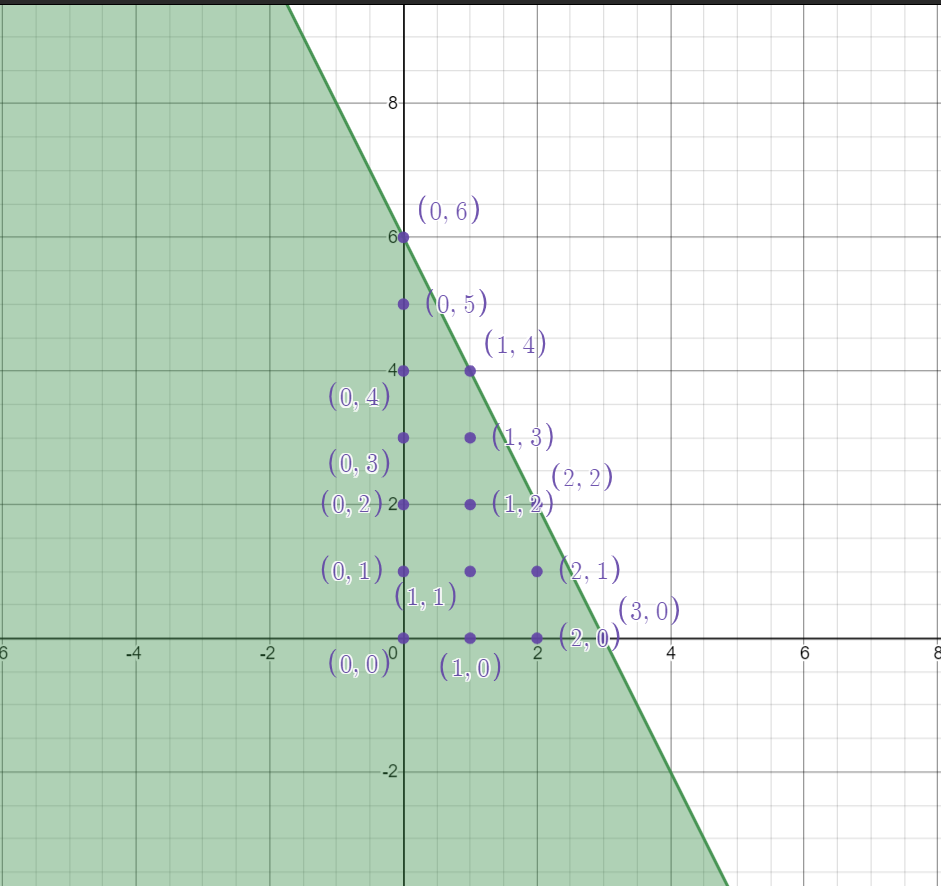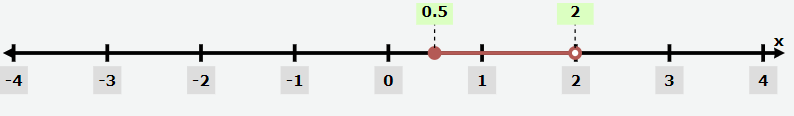#### Need Help?

Get in touch with us# Solving Inequalities – Explanation & Examples

In the context of Algebra, inequality can be defined as a mathematical statement that shows the relation between two expressions using the inequality symbols “>”, “<”, “≥”, and “≤”. The expressions on both sides of an inequality sign are not equal. It means that the algebraic expression on the left-hand side should be greater than or less than the expression on the right-hand side or vice versa.

Open Sentence: An inequality that contains only one variable.

Example: x < 10

Double Inequalities: An inequality representing the double relation of the number/expression.

Example: -5≤x<12

Strict Inequality

The less than the symbol “<” and the greater than the symbol “>” are called strict inequalities as they show that the numbers/expressions are strictly greater than or less than one another.

Example: 5 < 10

Slack Inequality

The less than or equal to symbol “≤” and the greater than or equal to symbol “≥” are called slack inequalities as they represent that the relation between the numbers/expressions is not a strict one. The final solution includes the boundary terms.

Example: x ≥ 15

## Properties of Inequalities

Before we start discussing solving inequalities, we must first understand some properties required for solving inequalities.

These properties also hold if all of the non-strict inequalities (≤ and ≥) are replaced by their corresponding strict inequalities (< and >).

In the case of applying a function, monotonic functions are limited to strictly monotonic functions.

#### Converse

The relations ≤ and ≥ are each other’s converse, meaning that for any real numbers a and b,

a ≤ b and b ≥ a is equivalent.

#### Transitivity

The transitive property of inequality states that for any real numbers a, b, and c, then if a ≤ b and b ≤ c, then a ≤ c.

If either of the premises is a strict inequality, then the conclusion is a strict inequality.

If a ≤ b and b < c, then a < c.

If a < b and b c, then a < c.

The addition or subtraction of a common constant c from both sides of the inequality does not affect the relationship. Inequality relation is preserved under addition and subtraction, and the real numbers are an ordered group under addition.

Let a, b, and c be real numbers.

If a≤b, then a+c≤b+c, and a-c≤b-c.

#### Multiplication and Division

The inequality relation is preserved under the multiplication and division operation with a positive constant. However, in the case of a negative constant, the inequality relation is reversed.

Let a and b be two real numbers, given that a ≤ b.

If c>0 and c≠0, then ac ≤ bc and a/c ≤b/c.

If c<0 and c≠0, then ac ≥ bc and a/c≥b/c.

The property for the additive inverse states that for any real numbers a and b if a ≤ b, then −a ≥ −b.

#### Multiplicative Inverse

For positive numbers, the inequality relation between their multiplicative inverses is the reverse of that between the original numbers themselves. For any two non-zero real numbers a and b that are both positive/negative, if a≤b, then 1/a≥1/b.

If 0 < a ≤ b, then 1/a≥1/b > 0.

If a ≤ b < 0, then 0>1/a ≥1/b.

If a < 0 < b, then 1/a < 0 <1/b.

#### Applying a Function to Both Sides

The inequality relation remains valid on the application of a monotonically increasing function on both sides of the equation, provided that these expressions lie in the domain of the function. However, the application of a monotonically decreasing function reverses the inequality.

If the inequality is strict and the function is strictly monotonic, then the inequality remains strict. But if only one of the conditions is strict then the inequality becomes non-strict.

Raising both sides of inequality to a power n > 0 (−n < 0), when a and b are positive real numbers:

0 ≤ a ≤ b; 0 ≤ an≤ bn

0 ≤ a ≤ b; a-n≥ b-n ≥ 0

Taking the natural logarithm on both sides of an inequality, when a and b are positive real numbers:

0 < a ≤ b; ln(a) ≤ ln(b)

0 < a < b; ln(a)< ln(b)

Let us now proceed to the methods for solving inequalities.

## How to Solve Inequalities?

There are two kinds of inequalities. Let us study them one by one.

### How to Solve Inequalities in One Variable?

If the linear inequality contains only one variable, it is called linear inequality in one variable. So, here we need to find the value of the unknown variable.

Question: 7x+3<5x+9

Solution:

Subtract 5x on both sides of the inequality

Thus,

7x+3-5x < 5x+9-5x

=2x+3 <9

=2x < 9-3

=2x < 6

=x < 3

Hence, the simplified form of the linear inequality 7x+3 < 5x+9 is x < 3.

If the linear inequality has only one variable, then the graph can be drawn using the number line. To graph the linear inequality, we must first solve for the given variable. After finding the solution set, we’ll use it to plot the graph of the given inequality. Let us take the example of the previous question i.e. 7x+3<5x+9.

The solution set for the inequality is x <3, which is equal to (-∞, 3).

### How to Solve Inequalities in Two Variables?If the linear inequality contains two variables, then it is called linear inequality in two variables. Here, we have to find the solution set for the pair of values of x and y i.e. (x, y).

Question: 40x+20y ≤ 120

Solution:

First take the L.H.S of an equation, 40x+20y

Let x=0, we get

40x+20y = 40(0)+20y

= 20y

Hence, 20y ≤ 120

=y ≤ 6

Thus, if x=0, then y can take the values of 0, 1, 2, 3, 4, 5, and 6, i.e. (0, 0), (0, 1), (0, 2), (0, 3), (0, 4), (0, 5), (0,6). Similarly, if we take x = 1, 2, and 3, the possible solutions are: (1, 0), (1, 1), (1, 2), (1, 3), (1, 4), (2, 0), (2, 1), (2, 2), (3, 0).

The graph of the linear inequality in two variables should be on the Cartesian plane or X-Y coordinate plane. Let us consider the inequality problem of the previously solved example: 40x+20y ≤ 120.

To graphically represent this equation, first, consider the equation 40x+20y = 120 and draw its graph. Now, plot the points (0, 0), (0, 1), (0, 2), (0, 3), (0, 4), (0, 5), (0,6), (1, 0), (1, 1), (1, 2), (1, 3), (1,4), (2, 0), (2, 1), (2, 2), (3, 0) in the coordinate plane.### Solving Compound Inequalities

Before we move on to solving compound inequalities, let us define what they are. A compound inequality includes two inequalities in a single statement. For example: -5≤x<12 means that -5≤x and also x<12. For solving compound inequalities, we can separate them into two simple single inequality statements and solve them as we’ve done before.

Question:

3 ≤ 2x + 2 < 6

Solution:

The first step is to write two separate inequalities: 3 ≤ 2x + 2 and 2x + 2 < 6.

We solve them independently as follows.

3 ≤ 2x + 2 and 2x + 2 < 6

1 ≤ 2x and 2x < 4

12 ≤ x and x < 2

Then, we can rewrite the solution as a compound inequality, the same way the problem began.

12 ≤ x < 2

In interval notation, the solution is written as [ 1/2,2)### How to Solve Absolute Value Inequalities?

Before we answer the question of How to solve absolute value inequalities let us first understand what absolute value means.

The absolute value of a quantity is always a positive number or zero. It is often used to describe the distance between two numbers as it is always positive regardless of the direction. Absolute value inequalities are equations of the form:

|A| < B, |A| ≤ B, |A| > B, or |A| ≥ B

Now let us answer the question of How to solve absolute value inequalities. For solving absolute value inequalities we need to obtain a set of values that satisfies the problem. It will be an interval or a union of two intervals that includes a range of values.

#### The Important Formulas for Solving Absolute Value Inequalities

Given below are some useful formulas for solving absolute value inequalities.

For an algebraic expression X and k>0,

|x|<k is equivalent to –k < x<k.

|x|>k is equivalent to x<-k or x>k.

These statements also apply to |x|<=k and |x|>=k.

Question: Describe all values within a distance of 4 from the number 5.

Solution:

We need the distance between x and 5 to be less than or equal to 4. This can be represented by an absolute value symbol |x-5|≤4.

We need to write two inequalities as there are always two solutions to an absolute value equation.

x – 5 ≤ 4 and x – 5 ≥ -4

x ≤ 9 and x ≥ 1

If the solution set is x ≤ 9 and x ≥ 1, then the solution set is an interval including all real numbers between and including 1 and 9.

So |x – 5| ≤ 4 is equivalent to [1, 9] in interval notation.#### Related topics#### Matrix – Represent a Figure Using Matrices

Key Concepts Representing a Figure Using Matrices:  To represent a figure using a matrix, write the x-coordinates in the first row of the matrix and write the y-coordinates in the second row of the matrix.           Addition and Subtraction With Matrices:  To add or subtract matrices, you add or subtract corresponding elements. The matrices must have […]#### Find the Distance Between Any Two Points in the X-Y Plane

Let P(x_1, y_1 ) and Q(x_2, y_2 ) be any two points in a plane, as shown in the figure. Hence, the distance ‘d’ between the points P and Q is d = √(〖(x_2-x_1)〗^2+〖〖(y〗_2-y_1)〗^2 ). This is called the distance formula. Find the distance between two points A(4, 3) and B(8, 6). Solution: Compare these […]#### Write an Equation of a Circle

Distance Between Ant Two Points in the X-y Plane Let P( x1, y1 ) and Q( x2, y2) be any two points in a plane, as shown in the figure.  Hence, the distance ‘d’ between the points P and Q is  d =√(x2−x1)2+(y2−y1)2 This is called the distance formula.  Solution: Compare these points with ( […]